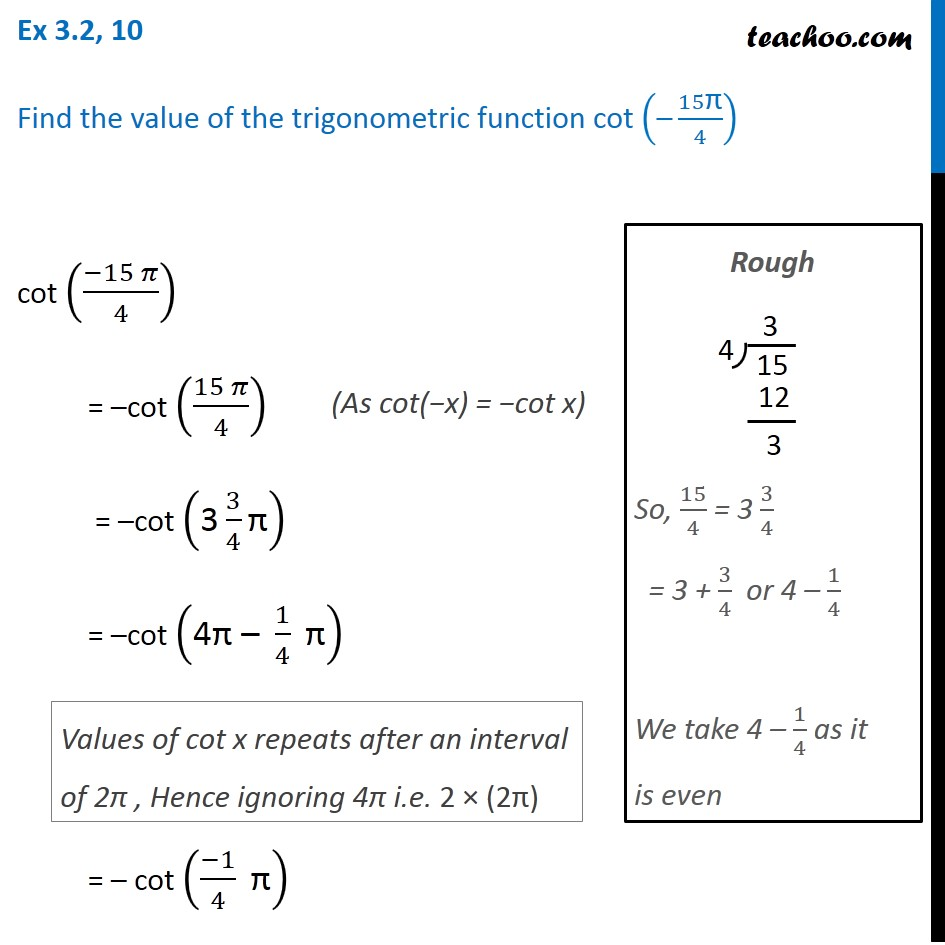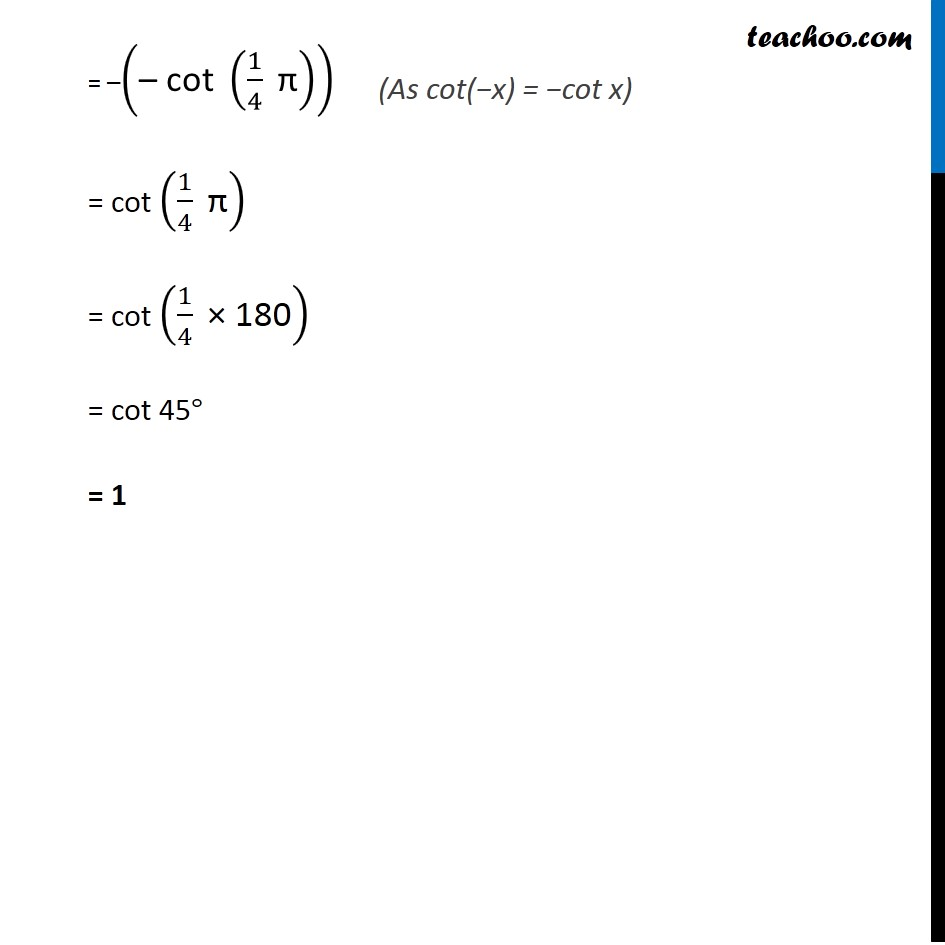Learn All Concepts of Chapter 2 Class 11 Relations and Function - FREE. Check - Trigonometry Class 11 - All Concepts1. Chapter 3 Class 11 Trigonometric Functions
2. Concept wise
3. Finding Value of trignometric functions, given angle

Transcript

Ex 3.2, 10 Find the value of the trigonometric function cot ("–" 15"π" /4) cot ((−15 𝜋)/4) = –cot ((15 𝜋)/4) = –cot ("3" 3/4 "π" ) = –cot ("4π – " 1/4 " π" ) = – cot ((−1)/4 " π" ) Values of cot x repeats after an interval of 2π , Hence ignoring 4π i.e. 2 × (2π) Rough So, 15/4 = 3 3/4 = 3 + 3/4 or 4 – 1/4 We take 4 – 1/4 as it is even = –("– cot " (1/4 " π" )) = cot (1/4 " π" ) = cot (1/4 " × 180" ) = cot 45° = 1

Finding Value of trignometric functions, given angle

About the AuthorDavneet Singh
Davneet Singh is a graduate from Indian Institute of Technology, Kanpur. He has been teaching from the past 9 years. He provides courses for Maths and Science at Teachoo.